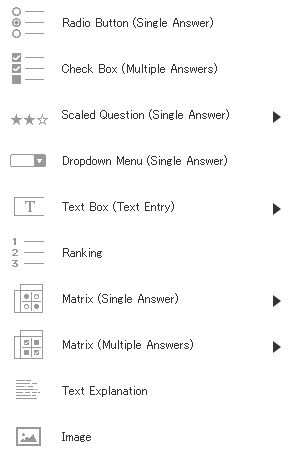# Question Types

Follow

There are 12 categories and 22 question types.

Sample Questionnaire:
https://questant.jp/q/sample_english● Radio Button (Choose Only One Answer)
Use this when only one answer can be selected.

● Checkbox (Choose Multiple Answers)
Use this when any number of answers can be selected.

● Scaled Question (Choose Only One Answer)
Use this when you want a rating level to be assigned.

● Drop-down Menu (Choose Only One Answer)
Use this when you want one answer chosen from specific choices.

● Text Box (Enter Text to Answer)
Use this when you want a free response, such as an opinion or impression.

● Ranking
Use this when you want the pull-down choices to be ranked in order.

● Matrix (Choose Only One Answer)
When multiple items are given the same answer choices, use this when only one answer　can be chosen for each question.

● Matrix (Choose Multiple Answers)
When multiple items are given the same answer choices, use this when multiple answers  can be chosen for each question.

Use this when a respondent add an image.

● Image
Use this when you want to show an image instead of giving answers to select.

● Text Explanation
Use this when you want to write an explanation text instead of giving answers to select.

● Video
Use this when you want to show a　movie file instead of giving answers to select.